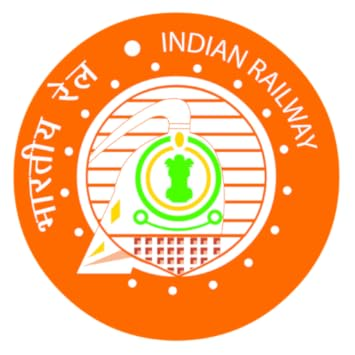### Quant Quiz

Q1. (9321+5406+1001) ÷ (498+929+660) =?
(a) 13.5
(b) 4.5
(c) 16.5
(d) 7.5
(e)18.5

Q2. 182.225×21.652×33.684 =?
(a) 136136
(b) 49428
(c) 120426
(d) 106438
(e) 84456

Q3. 63.9% of 8924.95 +? % of 5324.96 = 6827.9862
(a) 36
(b) 21
(c) 17
(d) 31
(e) 41

Q4. 40.02% of 601+ 250.17 =? – 75.01% of 911.98
(a) 1274
(b) 1147
(c) 1174
(d) 1074
(e) 1474

Q5. 20.06% of 599 + 10.01% of 901 =?
(a) 150
(b) 210
(c) 250
(d) 280
(e) 380

Solutions (1-5):

Q6. Rama’s expenditure and savings are in the ratio 5 : 3. If her income increases by 12% and expenditure by 15%, then by how much percent do her savings increase?
(a) 12%
(b) 7%
(c) 8%
(d) 13%
(e) 17%

Q7. The population of a town is 15000. If the number of males increases by 8% and that of females by 10%, then the population would increase to 16300. Find the number of females in the town.
(a) 4000
(b) 6000
(c) 3000
(d) 5000
(e) 5400

Q9. The marked price of an article is 50% above cost price. When marked price increased by 20% and selling price is increased by 20%, then profit doubles. If original marked price is Rs. 300, then original selling price is
(a) Rs. 200
(b) Rs. 250
(c) Rs. 240
(d) Rs. 275
(e) Rs. 320

Q10. A certain sum of money was invested at a certain rate of simple interest for 6 years, had it been invested at 3% higher rate, it would have yielded Rs.810 more. What was the principle that was invested?
(a) Rs. 27000
(b)  Rs. 18000
(c)  Rs. 1080
(d)  Rs. 4500
(e) Rs.  9000

Solutions (6-10):

Directions (11-15): The following line graph shows the production of pencils of two companies HB and Class mate during five different years. Study the graph carefully and answer the related questions.

Q11. What is the average no. of HB pencils produced throughout all the years?
(a) 48,900
(b) 44,800
(c) 46,800
(d) 46,200
(e) 44,650

Q12. If cost price of one class mate pencil in 2011 is Rs. 8 and class mate company made 75% profit as a whole in the same year then find the selling price of one class mate pencil.
(a) Rs. 16
(b) Rs. 14
(c) Rs. 12
(d) Rs. 10
(e) Rs. 18

Q13. The production of HB pencils in the years 2010, 2012 and 2014 together is approximately what percent of total classmate pencils produced in the years 2011, 2013 and 2014 together?
(a) 92%
(b) 72%
(c) 86%
(d) 80%
(e) Can’t be determined

Q14. If 10% class mate pencils out of total class mate pencils produced during all the years together found to be defective and company made a net profit of 20% on each pencil of the remaining non-defective pencils by selling at the rate of Rs.12 per piece then find overall profit/loss to the classmate company. (Production cost of pencil in every year is same)
(a) Rs. 1,98,400
(b) Rs. 1,94,400
(c) Rs. 1,89,400
(d) Rs. 1,96,400
(e) Rs. 1,94,800

Q15. What is the difference between total pencils produced by the two companies throughout all the years together?
(a) 16,000
(b) 14,000
(c) 12,500
(d) 14,400
(e) 16,600

## TOP COURSES

Courses offered by Us### BANKING

SBI/IBPS/RRB PO,Clerk,SO level Exams### SSC

WBSSC/CHSL/CGL /CPO/MTS etc..### RAILWAYS

NTPC/GROUP D/ ALP/JE etc..### TEACHING

REET/Super TET/ UTET/CTET/KVS /NVS etc..